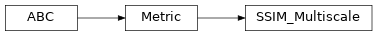# SSIM_Multiscale¶

Inheritance Diagramclass ashpy.metrics.ssim_multiscale.SSIM_Multiscale(name='SSIM_Multiscale', model_selection_operator=<built-in function lt>, logdir=PosixPath('/home/docs/checkouts/readthedocs.org/user_builds/ashpy/checkouts/latest/docs/source/log'), max_val=2.0, power_factors=None, filter_size=11, filter_sigma=1.5, k1=0.01, k2=0.03)[source]

Multiscale Structural Similarity.

See Multiscale structural similarity for image quality assessment 

  Multiscale structural similarity for image quality assessment https://ieeexplore.ieee.org/document/1292216

Methods

 __init__([name, model_selection_operator, …]) Initialize the Metric. split_batch(batch) Split a batch along axis 0 into two tensors having the same size. update_state(context) Update the internal state of the metric, using the information from the context object.

Attributes

 best_folder Retrieve the folder used to save the best model when doing model selection. best_model_sel_file Retrieve the path to JSON file containing the measured performance of the best model. logdir Retrieve the log directory. metric Retrieve the tf.keras.metrics.Metric object. model_selection_operator Retrieve the operator used for model selection. name Retrieve the metric name. sanitized_name all / are _.
__init__(name='SSIM_Multiscale', model_selection_operator=<built-in function lt>, logdir=PosixPath('/home/docs/checkouts/readthedocs.org/user_builds/ashpy/checkouts/latest/docs/source/log'), max_val=2.0, power_factors=None, filter_size=11, filter_sigma=1.5, k1=0.01, k2=0.03)[source]

Initialize the Metric.

Parameters: name (str) – Name of the metric. model_selection_operator (typing.Callable) – The operation that will be used when model_selection is triggered to compare the metrics, used by the update_state. Any typing.Callable behaving like an operator is accepted. Note Model selection is done ONLY if an operator is specified here. logdir (str) – Path to the log dir, defaults to a log folder in the current directory. max_val (float) – The dynamic range of the images (i.e., the difference between the maximum the and minimum) (see www.tensorflow.org/versions/r2.0/api_docs/python/tf/image/ssim_multiscale) power_factors (List[float]) – Iterable of weights for each of the scales. The number of scales used is the length of the list. Index 0 is the unscaled resolution’s weight and each increasing scale corresponds to the image being downsampled by 2. Defaults to (0.0448, 0.2856, 0.3001, 0.2363, 0.1333), which are the values obtained in the original paper. filter_size (int) – Default value 11 (size of gaussian filter). filter_sigma (float) – Default value 1.5 (width of gaussian filter). k1 (float) – Default value 0.01. k2 (float) – Default value 0.03 (SSIM is less sensitivity to K2 for lower values, so it would be better if we take the values in range of 0< K2 <0.4). None
static split_batch(batch)[source]

Split a batch along axis 0 into two tensors having the same size.

Parameters: batch (tf.Tensor) – A batch of images. Tuple[Tensor, Tensor] (Tuple[tf.Tensor, tf.Tensor]) The batch split in two tensors. ValueError – if the batch has size 1.
update_state(context)[source]

Update the internal state of the metric, using the information from the context object.

Parameters: context (ashpy.contexts.gan.GANContext) – An AshPy Context Object that carries all the information the Metric needs. None